# 06-240/Classnotes For Tuesday September 26

### Vector Spaces

Example 5.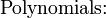$\mbox{Polynomials:}{}_{}^{}$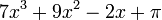$7x^3+9x^2-2x+\pi\$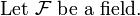$\mbox{Let } \mathcal{F }\ \mbox{be a field.}$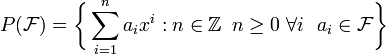$P(\mathcal{F})=\bigg\{ \sum_{i=1}^n a_i x^i :n \in \mathbb{Z}\,\ n\ge 0\ \forall i\ \ a_i \in \mathcal{F} \bigg\} {}_{}^{}$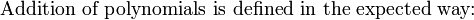$\mbox{Addition of polynomials is defined in the expected way:}{}_{}^{}$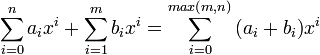$\sum_{i=0}^n a_i x^i + \sum_{i=1}^m b_i x^i =\sum_{i=0}^{max(m,n)}{(a_i+b_i)} x^i$

Theorem 1.(Cancellation law for vector spaces)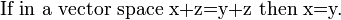$\mbox{If in a vector space x+z=y+z then x=y.}{}_{}^{}$

Proof: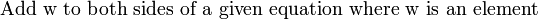$\mbox{Add w to both sides of a given equation where w is an element}{}_{}^{}$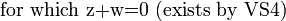$\mbox{for which z+w=0 (exists by VS4)}{}_{}^{}$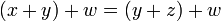$(x+y)+w=(y+z)+w \$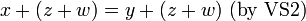$x+(z+w)=y+(z+w)\ \mbox{(by VS2)} {}_{}^{}$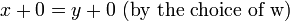$x+0=y+0\ \mbox{(by the choice of w)} {}_{}^{}$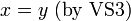$x=y\ \mbox{(by VS3)} {}_{}^{}$

Theorem 2. "0 is unique"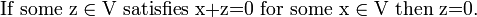$\mbox{If some z}\in\mbox{V satisfies x+z=0 for some x}\in \mbox{V then z=0.} {}_{}^{}$

Proof:"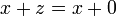$x+z=x+0\$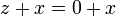$z+x=0+x\$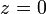$z=0\$

Theorem 3. "negatives are unique"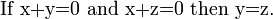$\mbox{If x+y=0 and x+z=0 then y=z.} {}_{}^{}$

Theorem 4.

a)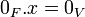$0_F.x=0_V\$

b)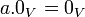$a.0_V=0_V\$

c)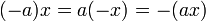$(-a)x=a(-x)=-(ax)\$

Theorem 5.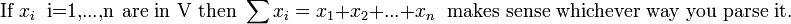$\mbox{If } x_i\ \mbox{ i=1,...,n are in V then } \sum {x_i}=x_1+x_2+...+x_n\ \mbox{ makes sense whichever way you parse it.} {}_{}^{}$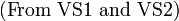$\mbox{(From VS1 and VS2)} {}_{}^{}$

### Subspaces

Definition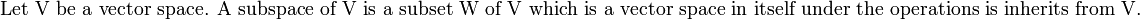$\mbox{Let V be a vector space. A subspace of V is a subset W of V which is a vector space in itself under the operations is inherits from V.}{}_{}^{}$

Theorem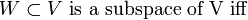$W\subset V\ \mbox{is a subspace of V iff}{}_{}^{}$

1.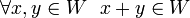$\forall x,y\in W\ \ x+y\in W \$
2.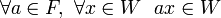$\forall a\in F,\ \forall x\in W\ \ ax\in W\$
3.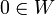$0 \in W\$

Proof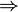$\Rightarrow$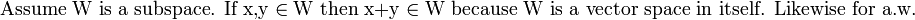$\mbox{Assume W is a subspace. If x,y} \in \mbox{W then x+y} \in \mbox{W because W is a vector space in itself. Likewise for a.w.}{}_{}^{}$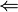$\Leftarrow$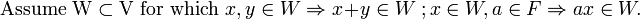$\mbox{Assume W}\subset \mbox{V for which } x,y\in W\Rightarrow x+y\in W\ ; x\in W, a\in F \Rightarrow ax\in W.\ {}_{}^{}$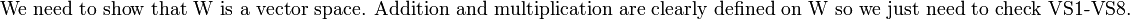$\mbox{We need to show that W is a vector space. Addition and multiplication are clearly defined on W so we just need to check VS1-VS8.}{}_{}^{}$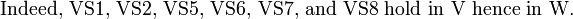$\mbox{Indeed, VS1, VS2, VS5, VS6, VS7, and VS8 hold in V hence in W.}{}_{}^{}$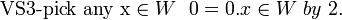$\mbox{VS3-pick any x}\in W\ \ 0=0.x\in W\ by\ 2.\ {}_{}^{}$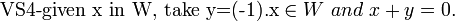$\mbox{VS4-given x in W, take y=(-1).x}\in W\ and\ x+y=0.\ {}_{}^{}$

Examples

Example 1.

Definition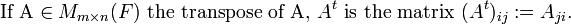$\mbox{If A}\in M_{m\times n}(F) \mbox{ the transpose of A, } A^t \mbox{ is the matrix } (A^t)_{ij}:=A_{ji}. {}_{}^{}$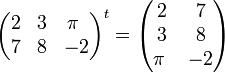$\begin{pmatrix} 2 & 3 & \pi\ \\ 7 & 8 & -2 \end{pmatrix}^t = \begin{pmatrix} 2 & 7 \\ 3 & 8 \\ \pi\ & -2 \end{pmatrix}$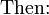$\mbox{Then:} {}_{}^{}$

1.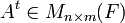$A^t \in M_{n\times m}(F)\$
2.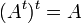$(A^t)^t=A\$
3.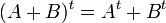$(A+B)^t=A^t+B^t\$
4.$(cA)^t=c(A^t)\ \forall c\in F\$

Definition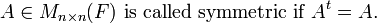$A\in M_{n\times n}(F) \mbox{ is called symmetric if } A^t=A. \ {}_{}^{}$

Claim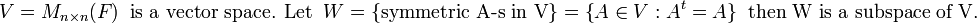$V=M_{n\times n}(F) \ \mbox{ is a vector space. Let } \ W=\big\{ \mbox{symmetric A-s in V}\big\} = \big\{ A\in V: A^t=A \big\}\ \mbox{ then W is a subspace of V.} {}_{}^{}$

Proof

1.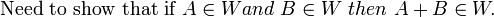$\mbox{Need to show that if } A\in W and\ B\in W\ then\ A+B\in W. \ {}_{}^{}$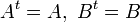$A^t=A,\ B^t=B \$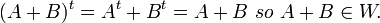$(A+B)^t=A^t+B^t=A+B\ so\ A+B\in W.$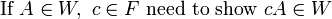$\mbox{If } A\in W,\ c\in F \mbox{ need to show } cA\in W {}_{}^{}$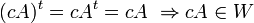$(cA)^t=cA^t=cA\ \Rightarrow cA\in W$

3.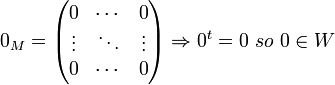$0_M=\begin{pmatrix} 0 & \cdots & 0 \\ \vdots & \ddots & \vdots \\ 0 & \cdots & 0\end{pmatrix} \Rightarrow 0^t=0 \ so \ 0\in W$

Example 2.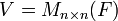$V=M_{n\times n}(F)$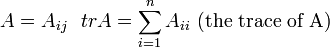$A=A_{ij}\ \ trA=\sum_{i=1}^n A_{ii}\ \mbox{(the trace of A)}$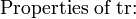$\mbox{Properties of tr:}{}_{}^{}$

1.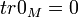$tr0_M=0 \$
2.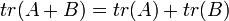$tr(A+B)=tr(A)+tr(B) \$
3.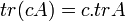$tr(cA)=c.trA \$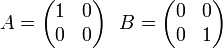$A=\begin{pmatrix} 1 & 0 \\ 0 & 0\end{pmatrix}\ \ B=\begin{pmatrix} 0 & 0 \\ 0 & 1\end{pmatrix} \$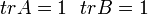$trA=1\ \ trB=1 \$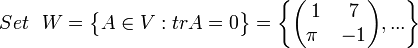$Set\ \ W=\big\{A\in V: trA=0\big\}=\bigg\{\begin{pmatrix} 1 & 7 \\ \pi\ & -1\end{pmatrix},...\bigg\} \$

Claim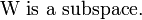$\mbox{W is a subspace.}{}_{}^{}$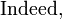$\mbox{Indeed,}{}_{}^{}$

1.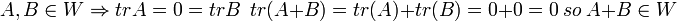$A,B\in W \Rightarrow trA=0=trB\ \ tr(A+B)=tr(A)+tr(B)=0+0=0\ so\ A+B\in W\$
2.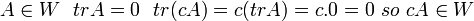$A\in W\ \ trA=0\ \ tr(cA)=c(trA)=c.0=0\ so\ cA\in W\$
3.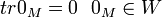$tr0_M=0\ \ 0_M\in W \$

Example 3.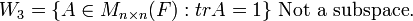$W_3=\big\{ A\in M_{n\times n}(F): trA=1\big\} \mbox{ Not a subspace.} {}_{}^{}$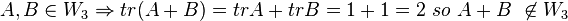$A,B\in W_3 \Rightarrow tr(A+B)=trA+trB=1+1=2\ so\ A+B\ \not\in W_3\$

Theorem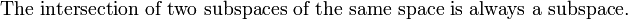$\mbox{The intersection of two subspaces of the same space is always a subspace.}{}_{}^{}$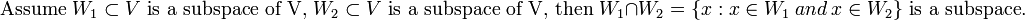$\mbox{Assume }W_1\subset V \mbox{ is a subspace of V, } W_2\subset V \mbox{ is a subspace of V, then }W_1\cap W_2=\big\{ x: x\in W_1 \ and\ x\in W_2\big\} \mbox{ is a subspace.}{}_{}^{}$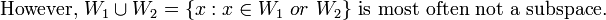$\mbox{However, }W_1\cup W_2=\big\{x: x\in W_1\ or\ W_2\big\} \mbox{ is most often not a subspace.} {}_{}^{}$

Proof

1.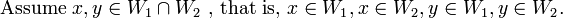$\mbox{Assume }x,y \in W_1\cap W_2 \mbox{ , that is, } x\in W_1, x\in W_2, y\in W_1, y\in W_2. \ {}_{}^{}$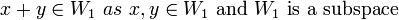$x+y\in W_1 \ as\ x,y\in W_1 \mbox{ and } W_1 \mbox{ is a subspace}{}_{}^{}$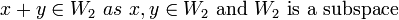$x+y\in W_2 \ as\ x,y\in W_2 \mbox{ and } W_2 \mbox{ is a subspace}{}_{}^{}$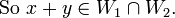$\mbox{So }x+y \in W_1\cap W_2. \ {}_{}^{}$

2.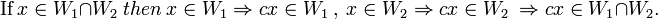$\mbox{If} \ x\in W_1\cap W_2\ then\ x\in W_1 \Rightarrow cx\in W_1\ ,\ x\in W_2 \Rightarrow cx\in W_2\ \Rightarrow cx\in W_1\cap W_2. \ {}_{}^{}$

3.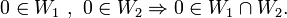$0 \in W_1\ ,\ 0\in W_2 \Rightarrow 0\in W_1\cap W_2. \$